# Advanced Curve Machining Method for 5-Axis CNC Machine Tools

A new method for curve machining on 5-axis CNC machine tools is proposed in this paper. It uses the CNC interpolator approach, or called curve interpolator, which can produce accurate tool position as well as tool orientation. The interpolator calculates a new command in real time, the same time period needed for sampling the control-loop feedback devices. It performs two steps in each sampling period: (1) trajectory planning based on a constant feedrate and (2) inverse kinematics transformation based on the structure of the machine. To implement this curve interpolator, a 3-D parametric curve g-code must be defined for 5-axis CNC machining. The comparison for the proposed method and the conventional off-line method in terms of trajectory accuracy feedrate variation are demonstrated in the end of this paper.

## INTRODUCTION

It has been known that 5-Axis CNC (Computer Numerical Control) machining for molds and dies produces higher metal removal rates and improved surface finish, compared with conventional 3-axis machining. However, the current methods for 5-axis machining utilize off-line part programming approaches by which the CAD system divides the surface into a set of line segments that approximates the surface at the desired tolerance. These line segments are further processed by post processors to produce straight-line g-codes which constitute the commands needed to control the machine. In the CNC system, these linear g-codes are fed into the interpolator that makes a linear motion for the curve. Chou and Yang provided a mathematical formulation for an Euler-angle-type 5-axis machine to track a parametric curve. Their work interpolated the curve correctly, but the required tool orientations were assumed to be given from CAD files.

This off-line approach for 5-axis machining either assumes a constant tool orientation along each segment, or assumes a linear change in the tool orientation between successive end-points. The constant orientation algorithm causes severe roughness around the end-points along the surface since the orientation changes are abrupt at these points. The linear orientation algorithm produces a better surface, but still cannot interpolate the orientations accurately between end points. This is because that the changes of the orientation along a curve are not necessarily linear and substantially causes machining errors. An additional drawback of the off-line methods is that the cutter accelerates and decelerates at each segment, which increases the surface non-uniformity and subsequently increases the cutting time.

To overcome these drawbacks, this paper proposes an algorithm for real-time 5-axis interpolator that generates both tool orientation and position precisely. This interpolator can produce desired trajectories for 5-axis curve machining. The interpolator calculates new commands for 5-axis controllers in the same time period, which is needed for sampling the control-loop feedback devices. The interpolator performs two steps in each sampling period: (1) trajectory planning based on a constant feedrate, and (2) inverse kinematics transformation based on the structure of the machine

The input to the interpolator is a new defined g-code (i.e., a CNC instruction), which contains the geometric information of the part surface as well as the cutting conditions, such as the feedrate, the spindle speed, the specific tool, etc. The interpolator begins with trajectory planning portion, which generates the position (x,y,z) and the orientation (Ox,Oy,Oz) of the cutter based on the curve geometry and the specified constant feedrate in each sampling period. This is a generic, machine independent algorithm. Based on the calculated cutter’s position and orientation, the reference values of the five axes can be obtained by the inverse kinematics transformation, an algorithm which depends upon the structure of each particular machine.

To use the proposed interpolator for 5-axis curve machining, new g-codes must be defined for programming the part program. In this paper, the 3-D parametric curve g-code for 5-axis machining will be given and illustrated by examples. The accuracy and feedrate analyses for the interpolating results will be discussed in the end of the paper.

## 5-AXIS CNC SYSTEMS

The motion control kernel of the CNC system consists of a real-time interpolator and a servo-controller that dominates the axis coordination and motion accuracy. Figure The proposed interpolator for 5-axis curve machining shows the structure of the CNC motion control kernel. As shown in the figure, the input to the CNC is the g-code type of part program. The major function of the real-time interpolator is to generate the reference commands for the servo-controller, which coordinates the multi-axis motion simultaneously.

The real-time interpolator for five-axis CNCs consists of the trajectory/ orientation planning module and the inverse kinematics transformation module. The trajectory/orientation planning generates the tool position (x,y,z) and orientation (Ox,Oy,Oz) based on the machining trajectory, or called tool path. The six (x,y,z,Ox,Oy,Oz) variables have to be transformed into five (X,Y,Z,A,B), which are the reference inputs for five servo-controllers. This transformation is called inverse kinematics transformation where the solution depends on the structure of a particular machine.

### A. Tool Trajectory

In order to produce smooth curves for a feed-drive CNC system, the machining feedrate (V) must be constant when the cutter tracks the desired trajectory. To keep the constant feedrate, the cutting tool has to move a constant distance relative to the workpiece in each sampling period of the interpolator. The desired tool trajectory can be expressed as a parametric curve C (u) and denoted as,

Applications of Computer Numerical Control (CNC) Machine in Manufacturing Industries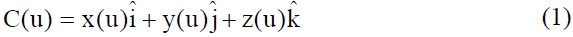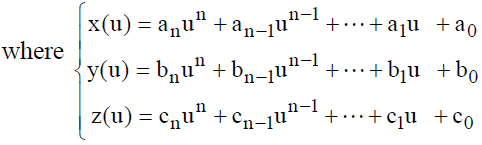Based on the parametric curve, the interpolator calculates the tool position at each sampling period (T). However, the constant change in the parameter u in the parametric domain does not guarantee the constant length in the Cartesian domain, and consequently does not guarantee a constant feedrate. To obtain the constant feedrate, the conversion between the parameter u and the constant distance that the tool moves at each sampling period has to be derived, A solution based on Taylor’s expansion is used to obtain the value of u that corresponds to equal trajectory length of the parametric curve.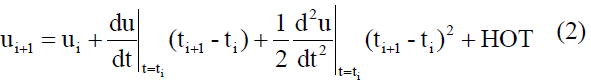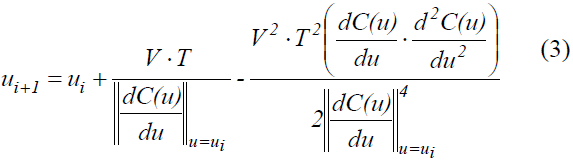This equation shows the function of u in terms of a constant length (VT), which is the distance that the tool moves during one sampling period T.

### B. Tool Orientation

5-Axis machine tools can control not only the tool position, but the tool orientation. The above tool trajectory planning calculates tool positions. The tool orientation can be obtained by calculating the main normal for the curve. The normal direction is perpendicular to the curve tangent. The unit tangent vector can be determined by,From the differential geometry, the rate change of tangent along the curve is the curve normal and denoted as,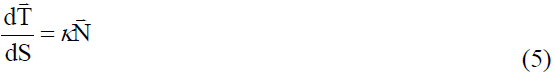where κ is the radius of curvature and Ñ is the unit normal vector. By rearranging the above equation, the unit normal can be obtained,### C. Inverse Kinematics

The above curve trajectory and normal represents the tool position(x,y,z) and orientation(Ox,Oy,Oz), respectively. However, the 5-axis machine tool has only 5 axes to be controlled. The transformation from the tool position and orientation into 5-axis reference positions uses inverse kinematics techniques.

The inverse kinematics transformation depends on the structure of the machine. The machine structure used for our derivations is shown in Fig. The structure of the horizontal five-axis machine tool, which is a horizontal 5-axis machine tool. As shown in Fig. The structure of the horizontal five-axis machine tool, two rotation axes are place on top of the Z-axis table. The tilting axis is rotating along the X axis, and denoted as A axis. The rotation axis is installed on top of the tilting axis and rotating along the Y axis, which denoted as B axis. In this case, the tool axis is fixed on the orientation [0,0,1]. To produce the part surfaces with tool orientation control on this five-axis machine, the part surface normal direction needs to be rotated into the fixed tool orientation [0,0,1].

Applications of Computer Numerical Control (CNC) Machine in Manufacturing Industries

Since the rotation axes are placed on top of the translation axes, the rotation and translation motions can be de-coupled. Therefore, the first step of the inverse kinematics transformation is to calculate the required rotation angles for tool orientations. We assume that the required tilting and rotating angles for this motion are A and B, respectively. A transformation equation with two rotations can be obtained in terms of the Homogeneous Transformation Matrices,

R(x, A) • R(y, B) • T [Ox ,Oy ,Oz ,1]T = [0,0,1,1](7)

where R(x, A) is the Homogeneous transformation matrix that rotates along the x axis with an angle A, shown in Eq. (8) in detail. Likewise, R(y, B) represents the notation of rotating along Y axis with an angle B. [0,0,1,1]T is the transpose matrix of [0,0,1,1], likewise T [Ox ,Oy ,Oz ,1] . The detailed expression for Equation (7) is shown below,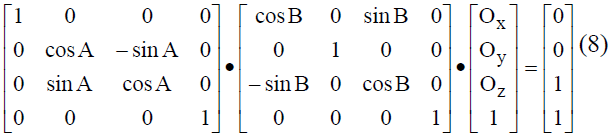Two unknowns, A and B, in Eq. (8) can be solved for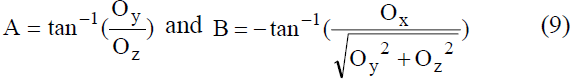After two simultaneously rotating motions, the tool position has been moved to a new location. Therefore, the next step is to find the distance between this tool location and the desired trajectory location. The determined distance in terms of XYZ coordinate system is the reference position for three translational axes. Using Homogeneous Transformation notation, this distance can be calculated as

[X, Y, Z, 1]T = T(OTx, OTy, OTz) • R(x,A) • T(Tx, Ty, Tz) • R(y, B) • T(Qx, Qy, Qz) • [x, y, z, 1]T   (10)

where T(a,b,c) represents a linear translation along a vector of [a,b,c] in the XYZ coordinate system. In Eq. (10) (x,y,z) is the tool location on the curve in terms of the part coordinate system. (Qx,Qy,Qz) is a relative distance between the part coordinate system and the rotating table (B-axis) coordinate system. (Tx,Ty,Tz) is a relative distance between the rotating coordinate system and the tilting table (A-axis) coordinate system. (OTx,OTy,OTz) is a relative distance between the tilting coordinate system to the tool coordinate system. Therefore, Equation (10) can be expressed as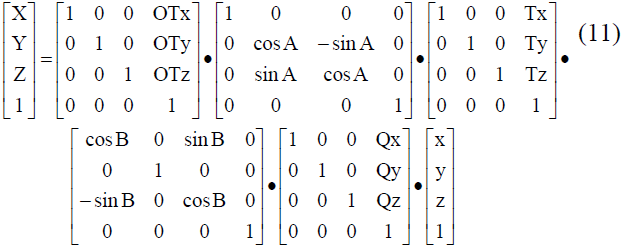The (X,Y,Z) in Eq. (11) and the (A,B) in Equation (9) are the solutions of inverse kinematics transformation , which are the reference commands for five-axis servo-controllers.

The controllers usually perform a specified control algorithm, e.g., PID control, to reduce the axial tracking errors.

## MOTION CODE

### A. Rational Bezier Curve

Free-form curves or surfaces have been widely used for product design in recent years. One of the convenient models for free-form curve is the parametric form. This rearch  uses rational Bezier curve, a parametric curve, for the CNC input format. A rational Bezier curve can be denoted as,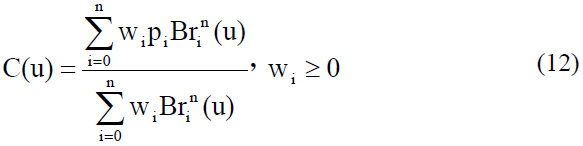where u is the parameter; p is the control point that controls the shape of the curve. Br is the blending function, which is a Bernstein Polynomial with order n-1. w is a wieght that adjusts the curve’s weighting.

### B. G-code Format

In order to descirbe a rational Bezier curve, the motion code, or called g-code, includes the control points and weights. A newly defined g-code format for the curve is shown below,

N01 G701 p1x p1y p1z w1 m
p2x p2y p2z w2
p3x p3y p3z w3
p4x p4y p4z w4

G701 is used for the curve interpoator motion word and N is the block number. The control point in the Cartisent Coordinated system is followed by the motion word, as well as the associated wieght. m is the number of control points to be used in this motion code. For this example, m is 4 and therefore, 3 more control points will be needed for this g-code.

An example is demonstrated for the above definition, as shown below.

N01 G701 X0     Y0    Z0    W.8 m4
X50   Y30  Z60  W.5
X80   Y80  Z30  W.2
X130 Y90  Z10  W.7

The result of the curve interpolation for this g-code is shown in Fig. The interpolating result of a rational Bezier curve

## RESULTS AND DISCUSSIONS

### A. Feedrate Analyses

The CNC system uses feed drive control technologies that control the motion by the specified program feedrate. Using the same sample above, the programmed feedrate is 3.6m/min (0.6m/sec) and the system sampling time is 0.01 sec. By making the time derivative of the axial position equation (X,Y,Z axes), the axial velocity can be obtained and shown in Fig. The velocities for 3 translational axes. Likewise, Figure The angular velocities for 2 rotating axes shows the angular velocities for the rotating axes (A and B).

In order to see the tool motion velocity, the distance for each sampling is calculated and shown in the Fig. The tool velocities during CNC motion. The velocity is between 0.5988 m/sec and 0.6002 m/sec, which keeps very close to the programmed feedrate 0.6m/sec. The calculation time for the curve interpolator for each iteration is
0.0044, which is less than the system sampling time and satisfies the CNC system regulation.

### B. Accuracy Analyses

The interpolating accuracy depends on the precision of the tool motion relative to the desired curve. The error between the these two trajectories is defined as the contour error. A scheme of the contour error is shown in the Fig. The contour error between the desired curve and the interpolated trajectory. By analyzing the contour errors for the developed curve interpolator, as shown in Fig. The contours for the interpolated results, the maximum contour error is 0.683mm and the average contour error is 0.118mm.

Applications of Computer Numerical Control (CNC) Machine in Manufacturing Industries

In contrast, the conventional curve machining uses off-line decomposition that causes large errors. With the same example above, it is decomposed into 21 line segments and subsequently feeds the 21 G01 motion codes into the CNC system. The contour errors for this machining method are shown in Fig. The contours for the conventional G01 method. The maximum error is 4.369mm. The error, in this machining method, can be reduced by increasing the number of decomposed line segments; however, it causes the longer machining time, as well as the mismatched tool orientations.